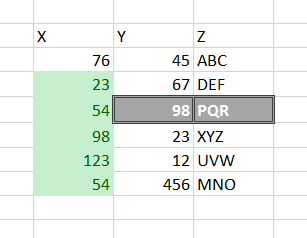# Multiply one cell value from one column with set of values of another column

Hello KNIME experts!

As I am pretty new to Knime, I am not sure if the below doubt has a direct solution:

As per sample data set:from this table I want to extract the Y value when Z is “PQR” and then multiply this Y value to all values of X (leave out value of X where Z is PQR) and then show these results to new columns.
so this result should be something like:

as you can see that for ‘n’ number of rows of X there will be n-1 new columns, each having one entry which is multiplication of one Y value(98) with all other X values (except the one where Z is “PQR”.

Major steps:

1. extract ‘PQR’ and attach Y-Value to the other rows
2. multiply
3. transpose

Z_031_multiply and pivot.knwf (17.2 KB)

Hope, that helps, br,
Tommy

1 Like

Hello @tommy ,

Thanks alot for your valuable suggestion…
This definitely worked for me and I could progress further.

Thanks!

2 Likes

This topic was automatically closed 7 days after the last reply. New replies are no longer allowed.